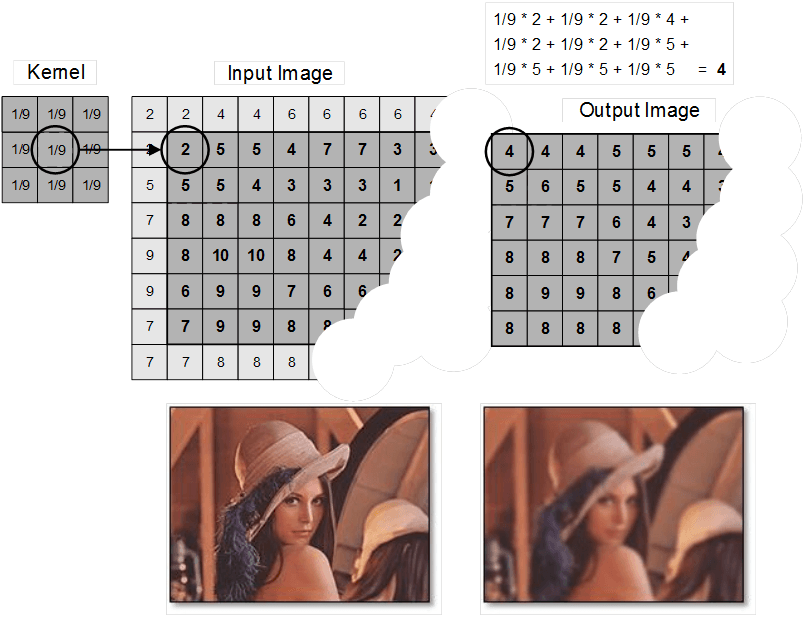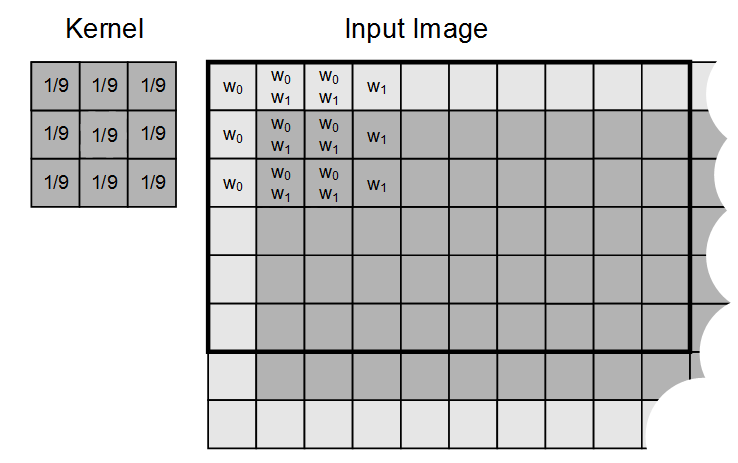## Heterogeneous compute case study: image convolution filtering

In a previously published article, I offered a quick guide to writing OpenCL kernels for PowerVR Rogue GPUs; this sets the scene for what follows next: a practical case study that analyzes image convolution kernels written using OpenCL.

Many image processing tasks such as blurring, sharpening and edge detection can be implemented by means of a convolution between an image and a matrix of numbers (or kernel). The figure below illustrates a 3×3 kernel that implements a smoothing filter, which replaces each pixel value in an image with the mean average value of its neighbours including itself.Kernel convolution usually requires values from pixels outside of the image boundaries. A variety of methods can be used to handle image edges, for example by extending the nearest border pixels to provide values for the convolutions (as shown above) or cropping pixels in the output image that would require values beyond the edge of an input image, which reduces the output image size.

The table below shows the algorithmic pseudo code for this filter on the left along with a C implementation on the right. In the C program, it is assumed that each pixel is represented by a 32-bit integer comprising four 8-bit values for R, G, B and A; the macro MUL4 therefore performs four separate multiplications, one for each of these 8-bit values.

 Convolution filter pseudo code Convolution filter in C ```void blur(int src[Gx][],int dst[Gx][], char *weight) { int x, y, pixel, acc;``` `  for each image row in input image:` `  for (y=1; y

By using the same approach as introduced in my previous article to extract the inner compute kernel from the serial control flow (the outer two nested loops), and applying the programming guidelines, the following OpenCL kernel is produced.

```__attribute__((reqd_work_group_size(8, 4, 1)))
__kernel void blur(image2d_t src, image2d_t dst, sampler_t s, float *weight)
{
int x = get_global_id(0);
int y = get_global_id(1);
float4 pixel = 0.0f;
for (j=-1; j<=1; j++) {
for (i=-1; i<=1; i++)
pixel += read_imagef(src, (int2)(x+i,y+j), s) * weight[j+1][i+1];
}
write_imagef(dst, (int2)(x,y), pixel/9.f);
}```

The statement

`__attribute__((reqd_work_group_size(8, 4, 1)))`

sets the workgroup size at compile-time to 32 (8x4). This restricts the host program to enqueuing this kernel as a 2D range with an 8×4 configuration, but improves the performance of the kernel when compiled.

The function declaration

`__kernel void blur(image2d_t src, image2d_t dst, sampler_t s, …`

specifies OpenCL image parameters and a sampler for accessing image data from system memory. To implement the border pixel behaviour in the above example, the host should configure the sampler as clamp-to-nearest-border (not shown).

The statement

`float4 pixel = 0.0f;`

defines a vector of four 32-bit floating point values. The threads use floating-point arithmetic to perform the convolution, which offers higher throughput compared to integer or character data types.

The statement

`read_imagef(src, s, (int2)(x+i,y+j))`

causes the TPU to sample a pixel from system memory into private memory, converting the constituent R, G, B and A values into four 32-bit floating-point values and placing these into a four-wide vector. This conversion is performed efficiently by the hardware, requiring the multiprocessor to issue just a single instruction.

The statement

`write_imagef(dst, (int2)(x,y), pixel/9.f);`

writes a (normalized) output pixel value back to system memory.

#### Caching frequently-used data in the common store

In the example in the previous section, all work-items operate independently of one another, each work-item independently sampling nine input pixels to calculate one output pixel. Overall the kernel has a fairly low arithmetic intensity (i.e. a low ratio of multiply-and-accumulate operations to memory sampling operations), which can lead to low performance.

For a workgroup size of 32, each workgroup performs a total of 288 (9×32) sampling operations. However, as show below, adjacent work-items use six of the same overlapping pixels from the input image.The common store is a fast on-chip memory that you can use to optimize access to frequently-used data, and also to share data between work-items in a workgroup, in this case enabling reduction of the number of sampling operations performed by a workgroup. A typical programming pattern is to stage data from system memory into common store by having each work-item in a workgroup:

• Load data from global memory to local memory.
• Synchronize with all other work-items in the workgroup, ensuring all work-items block until all memory reads have completed.
• Process the data in local memory.
• Synchronize with all other work-items in the workgroup, ensuring all work-items finish writing their results to local memory.
• Write the results back to global memory.

The example program below is a refinement of the previous program, rewritten to use local memory to reduce the number of sampling operations to system memory.

```__attribute__((reqd_work_group_size(8, 4, 1)))
__kernel void blur (image2d_t src, image2d_t dst, sampler_t s, float *weight)
{
int2 gid = (int2)(get_group(id(0)*8, get_group_id(1)*4);
int2 lid = (int2)(get_local_id(0),   get_local_id(1));
float4 pixel = 0.0f;

__local float4 rgb;
prefetch_texture_samples_8x4(src, sampler, rgb, gid, lid);

for (j=-1; j<=1; j++) {
for (i=-1; i<=1; i++)
pixel += rgb[lid.x+1+i][lid.y+1+i]) * weight[j+1][i+1]);
}
write_imagef(dst, (int2)(x, y), pixel/9.f);
}

void prefetch_texture_samples_8x4(image2d_t src, sampler_t s, __local float4 rgb , int2 gid, int2 lid)
{
if (lid.x == 0) {
// work-item 1 fetches all 60 rgb samples
for (int i=-1; i<9; i++) {
for (int j=-1; j<5; j++)
rgb[i+1][j+1] = read_imagef(src, s, gid+(int2)(i, j));
}
}
barrier(CLK_LOCAL_MEM_FENCE);
}```

The statement

`__local float4 rgb;`

declares a local array, which is allocated in the common store.

The kernel first calls the function

`void prefetch_texture_samples_8x4( …`

In this function, all work-items in a work-group first test their local ID together, and work-item 0 samples data from memory into the common store; all work-items then synchronize on a barrier. This synchronization operation is necessary to prevent the other work-items from attempting to read uninitialized data from the common-store. In the main kernel, calls to read_imagef are replaced by reads from the local memory array rgb.

In this optimized program each work-group performs a total of 60 sample operations, all during initialization, compared to the 288 in-line sampling operations performed in the original program. This reduction in memory bandwidth can significantly improve performance.

The prefetch function can be further improved so that instead of a single work-item fetching 60 samples in sequence, 30 work-items each fetch two samples in sequence. The following example shows one way in which this can be implemented.

```inline void prefetch_8x4_optimized(image2d_t src, sampler_t s, __local float4 rgb)
{
// Coord of wi0 in NRDange
int2 wi0Coord = (int2)(get_group_id(0)*8, get_group_id(1)*4);

// 2D to 1D address (from 8x4 to 32x1)
int flatLocal = get_local_id(1)*8 + get_local_id(0);

// Only first 30 work-items load, each loads 2 values in sequence
if (flatLocal < 30)
{
/* Convert from flatLocal 1D id to 2D, 10x3 */
int i = flatLocal % 10; // Width
int j = flatLocal / 10; // Height

/* 30 work iteams reads 10x3 values,
* values 0-9, 10-19, 20-29 from 10x6 - top half
*/
rgb[j][i] = read_imagef(src, s, (int2)(wi0Coord.x + i - 1, wi0Coord.y + j - 1));

/* 30 work iteams reads 10x3 values,
* values 30-39, 40-49, 50-59 from 10x6 - bottom half
*/
rgb[j + 3][i] = read_imagef(src, s, (int2)(wi0Coord.x + i - 1, wi0Coord.y + j + 3 - 1));
}
barrier(CLK_LOCAL_MEM_FENCE);
}```

In the best case, work-items can fetch data from the common store in a single cycle. In practice, however, a number of conditions must be met to achieve this efficiency.

Computer vision is what we’ll be focusing on for the next section of our heterogeneous compute series; stay tuned for an overview of how you build a computer vision platform for mobile and embedded devices.

Please let us know if you have any feedback on the materials published on the blog and leave a comment on what you’d like to see next. Make sure you also follow us on Twitter (@ImaginationTech) for more news and announcements from Imagination.